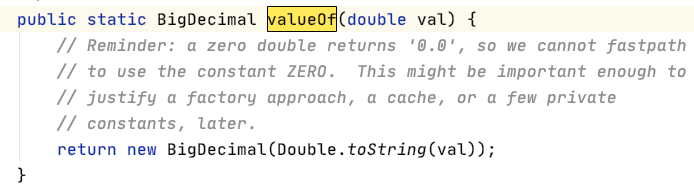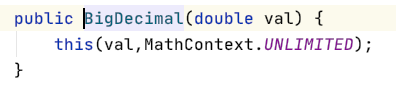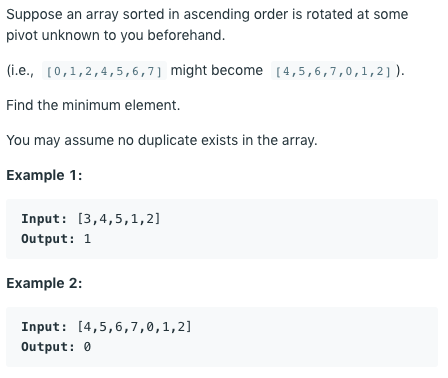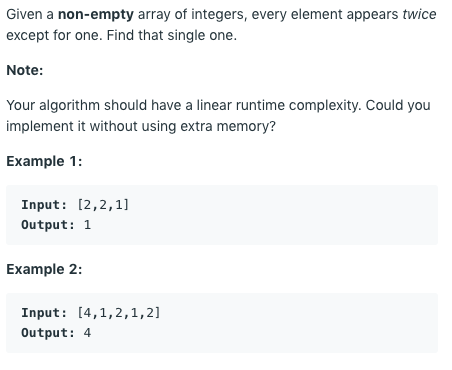## 字串轉數字

``````val numStr = "3.14"
val num0 = numStr.toDouble()
println(num0) // 3.14
``````

``````// val num10 = numStr.toInt() // 會發生 NumberFormatException
val num1 = numStr.toIntOrNull() ?: 0
println(num1) // 0
``````

``````val numStr2 = "abc"
val num2 = numStr2.toIntOrNull() ?: 0
val num3 = numStr2.toBigDecimalOrNull() ?: 0
println(num2) // 0
println(num3) // 0
``````

## 浮點數處理

``````val x = 10.1
val y = 5.53
val result = x - y
println(result) // 4.569999999999999
``````

``````println("%.2f".format(result)) // 4.57
``````

roundToInt() 則可以四捨五入取到整數

``````println(result.roundToInt()) // 5
``````

## BigDecimal

``````println("BigDecimal demo...")
println(BigDecimal.valueOf(x).minus(BigDecimal.valueOf(y))) // 4.57
println(BigDecimal.valueOf(x) - BigDecimal.valueOf(y))  // 4.57

println(BigDecimal.valueOf(x).plus(BigDecimal.valueOf(y))) // 15.63
println(BigDecimal.valueOf(x) + BigDecimal.valueOf(y)) // 15.63
``````

``````println(BigDecimal.valueOf(100.123).compareTo(BigDecimal.ZERO) > 0) // true
println(BigDecimal.valueOf(100.123) > BigDecimal.ZERO) // true
``````

``````println(BigDecimal(x) - BigDecimal(y)) //4.56999999999999939603867460391484200954437255859375
``````## 位元運算子 (bit operator)Java 中 右移運算子是 >>，左移運算子是 <<

## 為什麼要用位運算呢？

shl(1) 代表左移一位，也就是乘 2

shr(1) 代表右移一位，也就是除2

``````		// bit operator
println(42.shl(1)) // var43 = 42 << 1;  // 84
println(42.shr(1)) // var43 = 42 >> 1;  // 21
println(42 shr 1) // var43 = 42 >> 1;           // 21
``````

## Leetcode 153. Find Minimum in Rotated Sorted Array

https://leetcode.com/problems/find-minimum-in-rotated-sorted-array/``````fun findMin(nums: IntArray): Int {
var left = 0
var right = nums.size - 1

while (left < right) {
var mid = (left + right).shr(1)
if (nums[mid] > nums[right]) {
left = mid + 1
} else {
right = mid
}
}
return nums[left]
}
``````

`var mid = (left + right).shr(1)` 這裡取中間的 index 就使用了位運算

## Leetcode 136. Single Number

https://leetcode.com/problems/single-number/### 用位運算可以不用額外的空間！

2 xor 2 會變成 0010 xor 0010 = 0000 (因為 2 和 2 都一樣）

0 xor 5 會變成 0000 xor 0101 = 0101 結果還是 5

``````fun singleNumber(nums: IntArray): Int {
var result = 0;
nums.forEach {
result = result.xor(it); //do xor
}
return result;
}
``````